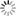# Episode List

OR

### Season 1

14 Sep. 1957
Marie WalewskaKnow what this is about?
Be the first one to add a plot.

23 Nov. 1957
Le sacrifice de Madame de LavaletteKnow what this is about?
Be the first one to add a plot.

18 Jan. 1958
L'orphelin de l'EuropeKnow what this is about?
Be the first one to add a plot.

25 Feb. 1958
Le mysérieux enlèvement du sénateur Clément de RisKnow what this is about?
Be the first one to add a plot.

25 Mar. 1958
L'exécution du duc d'EnghienKnow what this is about?
Be the first one to add a plot.

9 May 1958
L'étrange mort de Paul-Louis CourierKnow what this is about?
Be the first one to add a plot.

19 Oct. 1958
La mort de Marie-AntoinetteThe last hours of a deposed queen.

11 Nov. 1958
Il y a quarante ans...40 years after the November 1918 armistice, a flash back at World War I.

11 Jan. 1959
L'énigme de PiseKnow what this is about?
Be the first one to add a plot.

27 Feb. 1959
Le véritable AiglonKnow what this is about?
Be the first one to add a plot.

7 Jun. 1959
La dernière nuit de KoenigsmarkKnow what this is about?
Be the first one to add a plot.

20 Sep. 1959
La citoyenne VillirouëtKnow what this is about?
Be the first one to add a plot.

6 Feb. 1960
Le drame des poisonsKnow what this is about?
Be the first one to add a plot.

16 Apr. 1960
Qui a tué Henri IVKnow what this is about?
Be the first one to add a plot.

4 Jun. 1960
La nuit de VarennesKnow what this is about?
Be the first one to add a plot.

13 Dec. 1960
L'assassinat du duc de GuiseKnow what this is about?
Be the first one to add a plot.

11 Feb. 1961
L'énigme de Saint-LeuKnow what this is about?
Be the first one to add a plot.

22 Apr. 1961
Les Templiers
8.4 (7)Know what this is about?
Be the first one to add a plot.

24 Jun. 1961
Le drame de Sainte-HélèneKnow what this is about?
Be the first one to add a plot.

3 Oct. 1961
L'aventure de la duchesse de BerryKnow what this is about?
Be the first one to add a plot.

9 Dec. 1961
Le meurtre de Pierre IIIKnow what this is about?
Be the first one to add a plot.

17 Feb. 1962
L'affaire du collier de la reineKnow what this is about?
Be the first one to add a plot.

12 May 1962
Le meurtre de Henry Darnley ou La double passion de Marie StuartKnow what this is about?
Be the first one to add a plot.

11 Sep. 1962
Un meurtre sous Louis-PhilippeKnow what this is about?
Be the first one to add a plot.

8 Dec. 1962
La conjuration de Cinq-MarsThe story of the conspiration fomented by the Marquis de Cinq-Mars, favorite of King Louis XIII, with his friend de Thou against the Cardinal de Richelieu. This leads to the ruthless repression instigated by the Cardinal against the conspirators who die by the guillotine.

12 Jan. 1963
L'affaire CalasKnow what this is about?
Be the first one to add a plot.

23 Feb. 1963
La conspiration du général Malet
9.0 (7)Know what this is about?
Be the first one to add a plot.

13 Jul. 1963
La vérité sur l'affaire du courrier de Lyon
9.2Know what this is about?
Be the first one to add a plot.

14 Jul. 1963
La vérité sur l'affaire du courrier de Lyon #2Know what this is about?
Be the first one to add a plot.

2 Nov. 1963
Le procès de Charles 1erKnow what this is about?
Be the first one to add a plot.

14 Jan. 1964
Mata HariKnow what this is about?
Be the first one to add a plot.

4 Apr. 1964
Le mystère de ChoisyKnow what this is about?
Be the first one to add a plot.

2 May 1964
Le drame de MayerlingKnow what this is about?
Be the first one to add a plot.

10 Oct. 1964
La terreur et la vertu - Première partie: Danton
8.7 (14)Know what this is about?
Be the first one to add a plot.

17 Oct. 1964
La terreur et la vertu - Deuxième partie: Robespierre
9.1 (17)Know what this is about?
Be the first one to add a plot.

22 Jun. 1965
L'affaire LedruKnow what this is about?
Be the first one to add a plot.

22 Mar. 1966
Les Cathares - La croisade
8.8 (8)Know what this is about?
Be the first one to add a plot.

29 Mar. 1966
Les Cathares - L'inquisition
9.2Know what this is about?
Be the first one to add a plot.

Season 1